## Minimal Surface

Minimal surfaces are defined as surfaces with zero Mean Curvature, and therefore satisfy Lagrange's EquationMinimal surfaces may also be characterized as surfaces of minimal Area for given boundary conditions. A Plane is a trivial Minimal Surface, and the first nontrivial examples (the Catenoid and Helicoid) were found by Meusnier in 1776 (Meusnier 1785).

Eulerproved that a minimal surface is planar Iff its Gaussian Curvature is zero at every point so that it is locally Saddle-shaped. The Existence of a solution to the general case was independently proven by Douglas (1931) and Radó (1933), although their analysis could not exclude the possibility of singularities. Osserman (1970) and Gulliver (1973) showed that a minimizing solution cannot have singularities.

The only known complete (boundaryless), embedded (no self-intersections) minimal surfaces of finite topology known for 200 years were the Catenoid, Helicoid, and Plane. Hoffman discovered a three-ended Genus 1 minimal embedded surface, and demonstrated the existence of an infinite number of such surfaces. A four-ended embedded minimal surface has also been found. L. Bers proved that any finite isolated Singularity of a single-valued parameterized minimal surface is removable.

A surface can be parameterized using a Isothermal Parameterization. Such a parameterization is minimal if the coordinate functions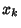are Harmonic, i.e.,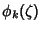are Analytic. A minimal surface can therefore be defined by a triple of Analytic Functions such that. The Real parameterization is then obtained as(1)

But, for an Analytic Function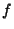and a Meromorphic function, the triple of functions(2)(3)(4)

are Analytic as long ashas a zero of orderat every Pole ofof order. This gives a minimal surface in terms of the Enneper-Weierstraß Parameterization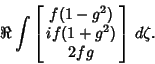(5)

See also Bernstein Minimal Surface Theorem, Calculus of Variations, Catalan's Surface, Catenoid, Costa Minimal Surface, Enneper-Weierstraß Parameterization, Flat Surface, Henneberg's Minimal Surface, Hoffman's Minimal Surface, Immersed Minimal Surface, Lichtenfels Surface, Maeder's Owl Minimal Surface, Nirenberg's Conjecture, Parameterization, Plateau's Problem, Scherk's Minimal Surfaces, Trinoid, Unduloid

References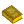Minimal Surfaces

Dickson, S. Minimal Surfaces.'' Mathematica J. 1, 38-40, 1990.

Dierkes, U.; Hildebrandt, S.; Küster, A.; and Wohlraub, O. Minimal Surfaces, 2 vols. Vol. 1: Boundary Value Problems. Vol. 2: Boundary Regularity. Springer-Verlag, 1992.

do Carmo, M. P. Minimal Surfaces.'' §3.5 in Mathematical Models from the Collections of Universities and Museums (Ed. G. Fischer). Braunschweig, Germany: Vieweg, pp. 41-43, 1986.

Douglas, J. Solution of the Problem of Plateau.'' Trans. Amer. Math. Soc. 33, 263-321, 1931.

Fischer, G. (Ed.). Plates 93 and 96 in Mathematische Modelle/Mathematical Models, Bildband/Photograph Volume. Braunschweig, Germany: Vieweg, pp. 89 and 96, 1986.

Gray, A. Modern Differential Geometry of Curves and Surfaces. Boca Raton, FL: CRC Press, p. 280, 1993.

Gulliver, R. Regularity of Minimizing Surfaces of Prescribed Mean Curvature.'' Ann. Math. 97, 275-305, 1973.

Hoffman, D. The Computer-Aided Discovery of New Embedded Minimal Surfaces.'' Math. Intell. 9, 8-21, 1987.

Hoffman, D. and Meeks, W. H. III. The Global Theory of Properly Embedded Minimal Surfaces. Amherst, MA: University of Massachusetts, 1987.

Lagrange. Essai d'une nouvelle méthode pour déterminer les maxima et les minima des formules intégrales indéfinies.'' 1776.

Meusnier, J. B. Mémoire sur la courbure des surfaces.'' Mém. des savans étrangers 10 (lu 1776), 477-510, 1785.

Nitsche, J. C. C. Introduction to Minimal Surfaces. Cambridge, England: Cambridge University Press, 1989.

Osserman, R. A Survey of Minimal Surfaces. New York: Van Nostrand Reinhold, 1969.

Osserman, R. A Proof of the Regularity Everywhere of the Classical Solution to Plateau's Problem.'' Ann. Math. 91, 550-569, 1970.

Radó, T. On the Problem of Plateau.'' Ergeben. d. Math. u. ihrer Grenzgebiete. Berlin: Springer-Verlag, 1933.